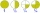# Division by zero

Fraction 5 by 2. if 3 is added to numerator and 2 is subtracted from the denominator then the new fraction is:

x =  INF

### Step-by-step explanation:Did you find an error or inaccuracy? Feel free to write us. Thank you!Tips to related online calculators
Need help to calculate sum, simplify or multiply fractions? Try our fraction calculator.

## Related math problems and questions:

• Denominator 2Denominator of a fraction is 5 and numerator is 7. Write the fraction .
• Guess a fractionTom was asked to guess a fraction. The sum of 1/2 the numerator and 1/3 of its denominator is 30. If Tom subtracts 36 from its denominator, the fraction becomes 1/3. What is the fraction that Tom was asked to guess? (Leave your answer in simplest form)
• Fractions and mixed numerals(a) Convert the following mixed numbers to improper fractions. i. 3 5/8 ii. 7 7/6 (b) Convert the following improper fraction to mixed number. i. 13/4 ii. 78/5 (c) Simplify these fractions to their lowest terms. i. 36/42 ii. 27/45 2. evaluate following ex
• Over fractionSolve equation: p over 5 equals fraction numerator 7 p over denominator 6 end fraction plus 5
• Quotient and productIf the quotient of [8/5 divided by 8/10] is added to the product of [8/14 x 7/12 x 3/8], what is the sum?
• Evaluate 11Multiply the quotient of 6 and 2 by 3, then add 1. Add 1 and 6, then divide by 2 and multiply by 3. Divide 6 by the product of 2 and 3, then add 1.
• Quotient 3If the quotient of 8/13 and 2 is subtracted from the product of 1 3/4 and 8/21, what is the difference?
• Product of two fractionsProduct of two fractions is 9 3/5 . If one of the fraction is 9 3/7. Find the other fraction.
• Find unknown 2Find unknown denominator: 2/3 -5/? = 1/4
• Mixed numbersRewrite mixed numbers, so the fractions have the same denominator: 5 1/5 - 2 2/3
• Fraction to decimalWrite the fraction 3/22 as a decimal.
• LCD 2The least common denominator of 2/5, 1/2, and 3/4
• Sum of fractionsWhat is the sum of 2/3+3/5?
• Product increasedWhen the product of 2/3 and 6/10 is increased by 2/5, the result is?3 3/4 + 2 3/5 + 5 1/2 Show your solution.If the temperature of a city drops 2° everyday and increases by 3° on every 5 days, how long does it take to drop to -15° from temperature +5°?I think of a fraction, if we increase its numerator and denominator by one, the value of the fraction increases by one tenth, what fraction do I think?